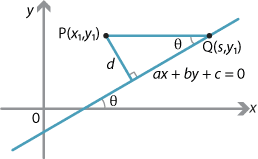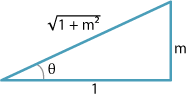## Content

### Perpendicular distances

Given a line $$l$$ and a point $$P$$ not on the line, it is natural to ask the question: What is the distance $$d$$ of $$P$$ from $$l$$?Detailed description

Assume that $$l$$ has equation $$ax+by+c=0$$, with $$a\ne0$$ and $$b\ne 0$$. We will also assume that the gradient $$m$$ of $$l$$ is positive. So the angle $$\theta$$ between $$l$$ and the positive $$x$$-axis is acute.

Let $$P(x_1,y_1)$$ be any point, and let $$Q(s,y_1)$$ be the point where the line $$l$$ meets the line through $$P$$ parallel to the $$x$$-axis. Since $$Q$$ lies on the line $$l$$, we have $$as+by_1+c=0$$ and so

$s = \dfrac{-by_1-c}{a}.$

Thus

$PQ = |x_1-s| = \Bigl|\dfrac{ax_1+by_1+c}{a}\Bigr|.$

We can draw the following triangle, as $$m=\tan\theta$$ with $$\theta$$ acute.Detailed description

From the triangle, we have

$\sin\theta = \dfrac{m}{\sqrt{1+m^2}}.$

Since $$m = -\dfrac{a}{b}$$, this implies that

$\sin^2\theta = \dfrac{m^2}{1+m^2} = \dfrac{a^2}{a^2+b^2}.$

We are assuming that $$\theta$$ is acute, so $$\sin \theta$$ is positive and therefore

$\sin\theta = \dfrac{|a|}{\sqrt{a^2+b^2}}.$

From the first diagram, we now have

$d = PQ\,\sin\theta = \dfrac{|ax_1+by_1+c|}{\sqrt{a^2+b^2}}.$
###### Theorem

Let $$P(x_1,y_1)$$ be a point and let $$l$$ be the line with equation $$ax+by+c=0$$. Then the distance $$d$$ from $$P$$ to $$l$$ is given by

$d = \dfrac{|ax_1+by_1+c|}{\sqrt{a^2+b^2}}.$
###### Proof

We have checked that the formula holds when $$a \ne 0$$, $$b \ne 0$$ and $$m > 0$$. Check that the formula also holds when $$a=0$$, when $$b=0$$ and when $$m < 0$$.

$$\Box$$

#### Example

What is the distance $$d$$ of the point $$P(-6,-7)$$ from the line $$l$$ with equation $$3x+4y=11$$?

#### Solution

Here $$a=3$$, $$b=4$$, $$c=-11$$, $$x_1=-6$$ and $$y_1=-7$$. So

$d = \dfrac{\bigl|-18-28-11\bigr|}{\sqrt{3^2+4^2}} = \dfrac{57}{5}.$

Exercise 9

A circle has centre $$(1,2)$$ and radius $$\sqrt{5}$$.

1. Find the perpendicular distance from the centre of the circle to the line with equation $$x+2y-10=0$$ and hence show this line is a tangent to the circle.
2. Find the perpendicular distance from the centre of the circle to the line with equation $$x+2y-12=0$$ and hence show the line does not meet the circle.

Exercise 10

Consider a line $$l$$ with equation $$ax+by+c=0$$. Show that the expression $$ax_1+by_1+c$$ is positive for $$(x_1,y_1)$$ on one side of the line $$l$$ and negative for all points on the other side.

Next page - Content - Geometrical proofs using coordinate methods